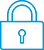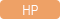cancel
Showing results for
Search instead for
Did you mean:It has been a while since anyone has replied. Simply ask a new question if you would like to start the discussion again.New member
2 1 0 0
Message 1 of 3
319
Flag Post

# Program with multiple inputs and CAS.SolveHP Recommended
HP Prime
Other

I want to create a program which solves a system of equations and calculates certain parameters depending on this solution.

I am having A LOT of trouble with the input. In the basic case, I want to have 9 inputs: a, b, c, d, e, ash, cP, wA and Hf. So far, what I've done is:

Spoiler

EXPORT comb_id()

BEGIN

LOCAL a, b, c, d, e, ash, cP, wA, Hf, gA, gHH, gH9H, gHs, porCO2hum, porCO2sec;

INPUT({{a, },{b, },{c, },{d, },{e, },{ash, },{cP, },{wA, },{Hf, }}, "Enter values", {"a", "b", "c", "d", "e", "ash", "C'", "wA", "H2Of"}, {"a", "b", "c", "d", "e", "ash", "C'", "wA", "H2Of"}, {0,0,0,0,0,0,0,0,0}, {0,0,0,0,0,0,0,0,0});

R = CAS.solve([a/12=beta, b/2=gamma, c/32+alphaQ=beta+gamma/2+delta, d/28+3.76*alphaQ=mu, e/32=delta], [alphaQ, beta, gamma, delta, mu])(1);

PRINT("alphaQ= " + R(1));

PRINT("beta= " + R(2));

PRINT("gamma= " + R(3));

PRINT("delta= " + R(4));

PRINT("mu= " + R);

gA = 32/.23*R(1);

PRINT("Air = " + gA);

gHH = gA + 1 - ash - cP;

gH9H = gHH - Hf - wA*gA;

gHs = gH9H - 9H;

gHs = 1;

porCO2hum = R(2) / (R(2) + R(3) + R(4) + R(5));

porCO2sec = R(2) / (R(2) + R(4) + R(5));

RETURN 1; //In fact, I want to return a vector with R and the other calculated variables concatenated.

END;

What I've also tried:

• Having variables declared as BEFORE the function name.
• Many, many, many, many other INPUT syntaxes. None work.

All the variables are real numbers,

How can I have a menu with these 9 inputs and have their default values be 0?

Also, for the more advanced case, I would like to have 3 radial buttons (can select only one) and then have the input screen depending on the user's radial selection. How can I make a radial button and grab its value?

I'm on software version 2.1.14181 (2019 10 16) and have hardware version A.

Thanks a ton!!!

Tags (2)
2 REPLIES 2Level 8
747 744 121 187
Message 2 of 3
Flag PostHP Recommended

You can only have 8 locals per line, and you can initialize with 0.

Local a:=0,b:=0, <up to 8 total>;

Local ghh:=0, <up to 8>;

Give that a go, 🙂

TW

Although I work for the HP calculator group as a head developer of the HP Prime, the views and opinions I post here are my own.New member
2 1 0 0
Message 3 of 3
Flag PostHP Recommended

This is what did the trick for me. I had to initialize the variables with a number for some reason.

Regarding CAS.solve, I tried using

Spoiler
LOCAL cmd := "solve([" + eqns + ", " + unknowns + "])"
LOCAL result := CAS(cmd);

To get a result, but the variable result only contained the command as a string (note that eqns and unknowns are both strings with the equations I wanted to solve).

To my luck, my system has a closed form solution, so I basically solved every case in Mathematica and now this program just spits that result.

Thanks!!

† The opinions expressed above are the personal opinions of the authors, not of HP. By using this site, you accept the Terms of Use and Rules of Participation